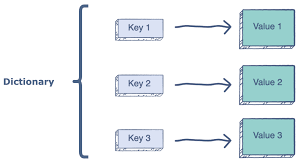×

Search anything:

# Different Dictionaries in Python

#### Software Engineering PythonDictionary is a collection of items where each item is a Key:Value pair.The values can be repeated but the keys must be unique.Each value and key can be of any type.

There are 4 types of dictionaries in Python:

• OrderedDict
• DefaultDict
• ChainMap
• CounterPython code for dictionary

``````new_dict=dict()
new_dict['a']="apple"
new_dict['b']="ballon"
print(new_dict['a'])
``````

Output: apple

Dictionaries are hashed and stored which means accessing the elements within the dictionary is very fast i.e O(1).

We can also use get() method to get the value of given key:

``````new_dict=dict()
new_dict['a']="apple"
new_dict['b']="ballon"
print(new_dict.get('b'))
``````

Output: ballon

Accessing elements using items() method:

``````new_dict=dict()
new_dict['a']="apple"
new_dict['b']="ballon"
new_dict['c']="car"
for key,value in new_dict.items():
print(key,value)
``````

Output:
b ballon
a apple
c car

Accessing element which is not present in dictionary generates error

``````print(new_dict['d'])
``````

Output: KeyError: 'd'

### Methods in dictionary:

• copy() : returns a shallow copy
• clear() : clears the dictionary
• pop() : removes and returns the element with given key
• popitem() : removes and returns the a random element as a tuple
• get() : gets the value of the given key
• values() : returns all values present as an list
• str() : returns string representation of dictionary
• update() : updates the dic1 with that of dic2's value
• setdefault() : sets default value
• keys() : returns all keys as a list
• items() : returns key-value pair as a tuple
• has_key() : returns true if it has given key else false
• type() : returns type
• cmp() : compares the elements of both dictionaries
• fromkeys() : generates a dictionary from given keys with same value

### Types of Dictionaries:

There are 4 types of dictionaries in Python:

• OrderedDict
• DefaultDict
• ChainMap
• Counter

#### OrderedDict:

OrderedDict is a subclass of dictionary class which maintains the elements in the inserted order.Normally Dictionary does not preserve the inserted order.It is present in collections library so it must be imported before using it otherwise it generates error.

``````from collections import OrderedDict
new_dic=OrderedDict()
new_dic['a']="apple"
new_dic['b']="banana"
new_dic['c']="carrot"

for key,value in new_dic.items():
print(key,value)
``````

Output:
a apple
b banana
c carrot

As we can observe from the output that the order of output is same as that of insertion.

Methods:

• popitem(last): It returns and removes a (key,value) pair. The pairs are in Last In First Out order if last is True else it would be in First In First Out order if last is False.
``````from collections import OrderedDict
new_dict=OrderedDict()
new_dict['a']=1
new_dict['b']=2
new_dict['c']=3
print(new_dict.popitem(last=True))
``````

Output:('c', 3)

• move_to_end(key,last): It moves the given key to the end of the ordered dictionary. It moves to right end if last is true else it moves to the beginning.It raises KeyError when given is not present in the orderedDict.
``````from collections import OrderedDict
new_dict=OrderedDict()
new_dict['a']=1
new_dict['b']=2
new_dict['c']=3
new_dict.move_to_end('a',True)
print(new_dict.keys())
``````

Output: KeysView(OrderedDict([('b', 2), ('c', 3), ('a', 1)]))

#### DefaultDict

Dictionary in python generate error when we try to access the key which is not present in the dictionary. This error(KeyError) stops the execution of the program.DefaultDict is best solution for the above problem.It is present in collections library so it must be imported before using it otherwise it generates error.

Unlike normal dictionary ,defaultdict does not raise KeyError. It provides a default value for the key which is not present in the dictionary.A function should be passed which should return a string to determine when key is not present in the dictionary.
The function which is to be passed is called as default_factory.

``````from collections import defaultdict
def def_value():
return "Not existing"
new_dic=defaultdict(def_value)
new_dic="hello"
print(new_dic)
``````

Output: Not existing

#### ChainMap:

It is a container which combines multiple dictionaries into single unit.It is present in collections library.

``````from collections import ChainMap
a={1:"Hi",2:"opengenus"}
b={'g':"my",'h':"first blog"}
c=ChainMap(a,b)
print(c)
``````

Output: ChainMap({1: 'Hi', 2: 'opengenus'}, {'h': 'first blog', 'g': 'my'})

Methods:

• maps() : It returns the corresponding keys along with values of corresponding dictionaries.
``````from collections import ChainMap
a={1:"Hi",2:"opengenus"}
b={'g':"my",'h':"first blog"}
c=ChainMap(a,b)
print(c.maps)
``````

Output: [{1: 'Hi', 2: 'opengenus'}, {'h': 'first blog', 'g': 'my'}]

• new_child(m) : It adds a new dictionary to the beginning of the ChainMap.It takes an optional parameter which when given gets added otherwise empty dictionary gets added to the beginning of the ChainMap.
``````from collections import ChainMap
a={1:"Hi",2:"opengenus"}
b={'g':"my",'h':"first blog"}
c=ChainMap(a,b)
c=c.new_child({'t':'Hello user'})
print(c)
``````

Output:
ChainMap({'t': 'Hello user'}, {1: 'Hi', 2: 'opengenus'}, {'g': 'my', 'h': 'first blog'})

• reversed() : It reverses the order of dictionaries in the ChainMap.
``````from collections import ChainMap
a={1:"Hi",2:"opengenus"}
b={'g':"my",'h':"first blog"}
c=ChainMap(a,b)
print("Before reversed ",c)
c.maps=reversed(c.maps)
print("After reversed ",c)
``````

Output:
Before reversed ChainMap({1: 'Hi', 2: 'opengenus'}, {'h': 'first blog', 'g': 'my'})
After reversed ChainMap({'h': 'first blog', 'g': 'my'}, {1: 'Hi', 2: 'opengenus'})

## Question

#### Output of the following code

###### from collections import OrderedDict new_dict=OrderedDict() new_dict['a']=1 new_dict['b']=2 new_dict['c']=3 new_dict.move_to_end('b',False) print(new_dict.keys())
KeysView(OrderedDict([('a', 1), ('b', 2), ('c', 3)]))
KeysView(OrderedDict([('a', 1), ('c', 3), ('b', 2)]))
KeysView(OrderedDict([('b', 2), ('a', 1), ('c', 3)]))
KeysView(OrderedDict([('c', 3), ('a', 1), ('b', 2)]))
As False is given it should be moved to the beginning end. Normal order would be a,b,c but after moving to end order would be b,a,c

#### Counter:

It is a subclass of the dictionary class. It stores the count of each element present along with the element.

``````from collections import Counter
l=['a','b','a','c']
c=Counter(l)
print(c)
``````

Output:
Counter({'a': 2, 'b': 1, 'c': 1})

Methods:

• elements() : It returns an iterator over elements repeating the number of count of each. It does not count for count<=0.
``````from collections import Counter
c=Counter(a=4, b=2, c=0, d=-2)
print(list(c.elements()))
``````

Output:['b', 'b', 'a', 'a', 'a', 'a']

• most_common() : It returns the most common elements along with their counts. It takes optional parameter m which determines how many to number of items to return from highest count to lowest count. If m is not given then all are returned.
``````from collections import Counter
c=Counter(a=4, b=2, c=0, d=-2)
print(c.most_common(3))
``````

Output:[('a', 4), ('b', 2), ('c', 0)]